Many types of graphic objects use formulas to calculate what should be displayed by the object. For example a Text Display Object uses a formula to directly calculate the text that will be displayed. For other objects, like the Image Display Object, the calculated text itself isn’t displayed directly, but instead indirectly controls what is displayed. Some objects even have multiple formulas associated with the object, for example the Text List Object and Matrix Object have three formulas: a formula for the main display, a formula for the query, and a formula for the selected item.

By using formulas embedded in objects Panorama gives you tremendous flexibility and power to make your objects work as you need them to. However, what if your formula doesn’t calculate the value you expect, or what if your formula contains an error? If the object doesn’t display the formula directly, how can you diagnose what the problem is? Even a Text Display Object can make it difficult to diagnose if the object is too small to display the entire error message.

### The Object Value Dialog

The Object Value dialog is designed to help with diagnosing formula problems. This dialog displays the raw text that is calculated by the formula (or formulas) embedded in the selected object. (You must be in Graphics Mode to use this dialog.

For example, consider the form object below. The form contains a Web Browser Object that is intended to display a map by using the mapurl( function (this function converts an address into the URL necessary to display a map). The formula looks correct, but no map is being displayed.😢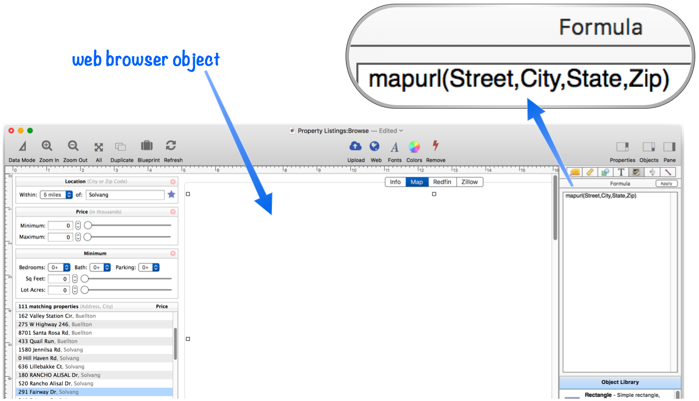Since the Web Browser Object doesn’t display the text, it’s not immediately obvious what is wrong with the formula. Fortunately I can use the Object Value dialog to see what the formula result is. To use this dialog, first select the object, then choose Object Value from the Objects menu. Alternately, select the object then right click and choose Object Value from the pop-up menu, as shown here.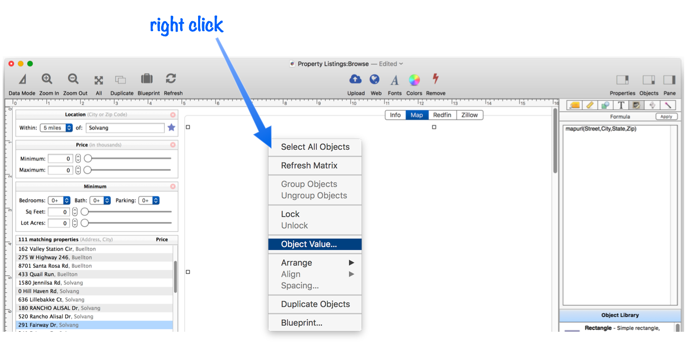The Object Value dialog shows the raw result of the formula, making it easy to see that the problem is that the formula uses the field name Street, when really the field name in this database is Address.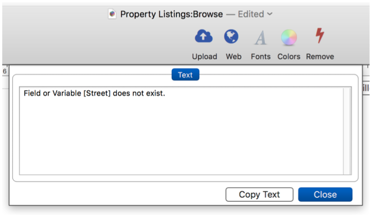When I correct the formula and press Apply, the map appears.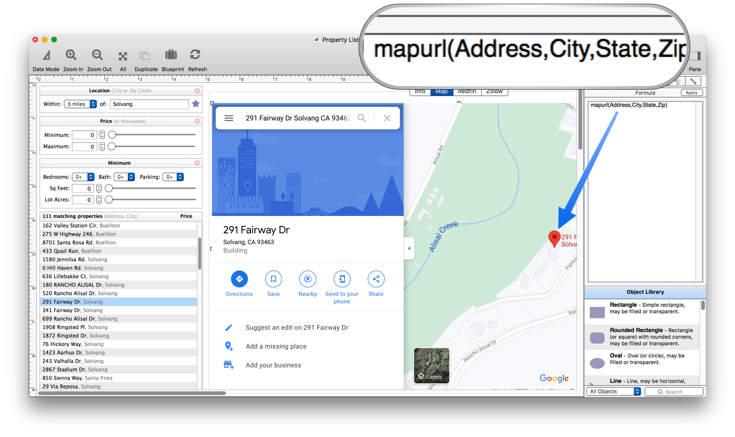If I now go back and display the Object Value again, the dialog will show the URL for the map that is being displayed. This is useful for verifying that the calculated result is correct (after all, just because there is no error does not mean the value is correct).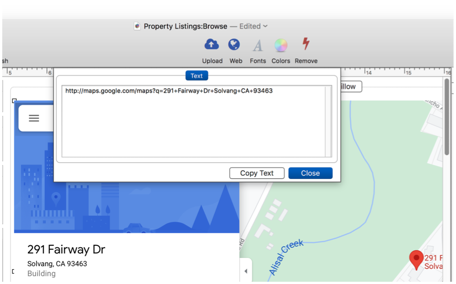#### Displaying Multiple Object Values

Some types of objects have multiple formulas embedded in them. For example a Text List Object contains three formulas, as shown in this diagram.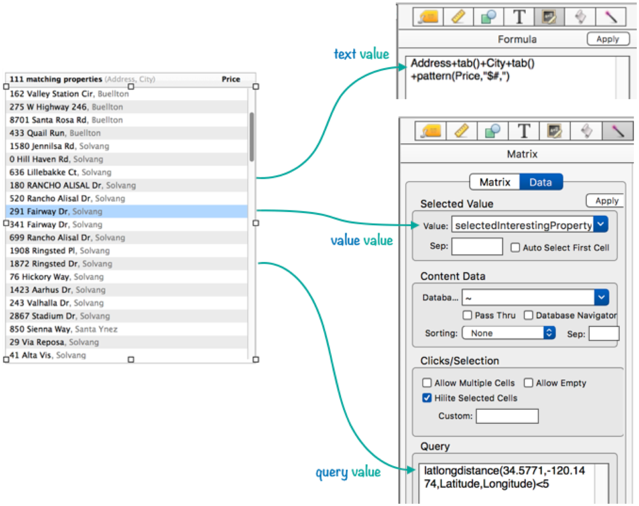When you open the Object Value dialog, Panorama automatically detects that there are three values available and allows you to view any of the values.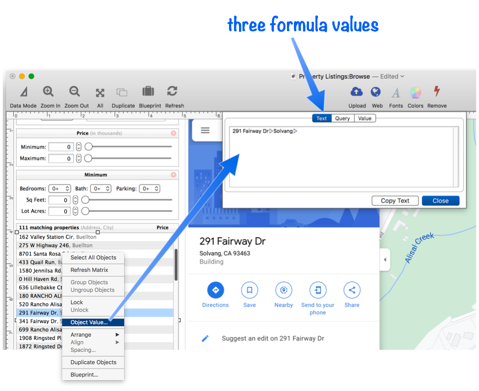#### Object Value Dialog Restrictions

The Object Value command is only available if there is a single object selected. If there are no objects selected, or two or more objects, the Object Value menu item will be disabled.

The Object Value command is also limited to objects that contain an embedded formula. If the selected object does not contain a formula (for example a basic shape object), the Object Value menu item will be disabled.